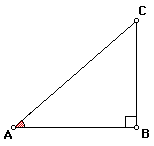# Trigonometry RatiosSine, cosine, and tangent are functions which compare an angle in a right triangle to the ratio of two of the sides. On a calculator they are marked sin, cos, and tan, respectively.

Using GSP, create and measure several right triangles (angles and sides). Find the sin(<CAB), cos(<CAB), and tan(<CAB), and determine which side ratios they are each equal to.

Repeat the process with <ACB and explain a general rule for determining the sine, cosine, and tangent of an angle in a right triangle.Extension
The slope of line AC is equivalent to which of these functions?